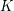Importance: Low ✭
 Author(s): Georgakopoulos, Agelos
 Subject: Graph Theory » Infinite Graphs
 Keywords: end ray
 Posted by: Agelos on: February 3rd, 2008
Problem   Letbe a graph,a countable end of, andan infinite set of pairwise disjoint-rays in. Prove that there is a setof pairwise disjoint-rays that devourssuch that the set of starting vertices of rays inequals the set of starting vertices of rays in.

We say that a set of raysdevours the endif every ray inmeets some ray in. An end is countable if there is a countable set of rays devouring it.

Ifis a finite set of rays then it is not hard to prove (see [G]) that this problem has a positive answer:

Theorem   For every graphand every countable endof, ifhas a setofpairwise disjoint-rays, then it also has a setofpairwise disjoint-rays that devours. Moreover,can be chosen so that its rays have the same starting vertices as the rays in~.

## Bibliography

*[G] A. Georgakopoulos, Infinite Hamilton Cycles in Squares of Locally Finite Graphs, Preprint.

* indicates original appearance(s) of problem.